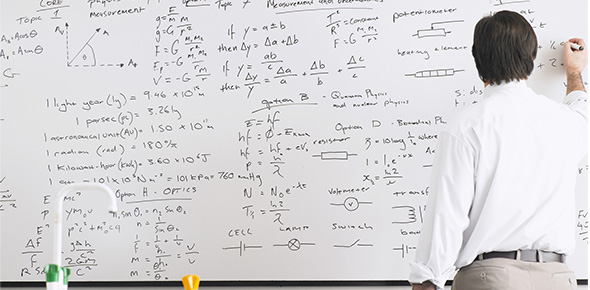9050 Difference Equation Terminology Rule & Ard

10 Questions | Total Attempts: 150Settings• 1.
In the Difference Equation above, what are the values for a, r and d? (comma/space separated : ie, 9, 7, 2)
• 2.
In the Difference Equation above, what are the values for a, r and d? (comma/space separated : ie, 9, 7, 2)
• 3.
In the Difference Equation above, what are the values for a, r and d? (comma/space separated : ie, 9, 7, 2)
• 4.
In the Difference Equation above, what are the values for a, r and d? (comma/space separated : ie, 9, 7, 2)
• 5.
In the Difference Equation above, what are the values for a, r and d? (comma/space separated : ie, 9, 7, 2)
• 6.
In the Difference Equation above, what are the values for a, r and d? (comma/space separated : ie, 9, 7, 2)
• 7.
In the Difference Equation above, what are the values for a, r and d? (comma/space separated : ie, 9, 7, 2)
• 8.
In the Difference Equation above, what are the values for a, r and d? (comma/space separated : ie, 9, 7, 2)
• 9.
In the Difference Equation above, what are the values for a, r and d? (comma/space separated : ie, 9, 7, 2)
• 10.
In the Difference Equation above, what are the values for a, r and d? (comma/space separated : ie, 9, 7, 2)
• 11.
In the Difference Equation above, what are the values for a, r and d? (comma/space separated : ie, 9, 7, 2)
• 12.
In the Difference Equation above, what are the values for a, r and d? (comma/space separated : ie, 9, 7, 2)
• 13.
In the Difference Equation above, what are the values for a, r and d? (comma/space separated : ie, 9, 7, 2)
• 14.
In the Difference Equation above, what are the values for a, r and d? (comma/space separated : ie, 9, 7, 2)
• 15.
In the Difference Equation above, what are the values for a, r and d? (comma/space separated : ie, 9, 7, 2)
• 16.
In the Difference Equation above, what are the values for a, r and d? (comma/space separated : ie, 9, 7, 2)
• 17.
Which statement best describes the image above?
• A.

This is a difference equation

• B.

This is a rule for a difference equation

• C.

This is the value for 'a'

• D.

None of the other statements are accurate

• 18.
Which statement best describes the image above?
• A.

This is a difference equation

• B.

This is a rule for a Difference Equation

• C.

This is the value for 'a'

• D.

None of the other statements are accurate

• 19.
Which statement best describes the image above?
• A.

This is a difference equation

• B.

This is a rule for a Difference Equation

• C.

This is the value for 'a'

• D.

None of the other statements are accurate

• 20.
Which statement best describes the image above?
• A.

This is a difference equation

• B.

This is a rule for a difference equation

• C.

This is a value for 'a'

• D.

None of the other statements are accurate

• 21.
Which statement best describes the image above?
• A.

This is a difference equation

• B.

This is a rule for a difference equation

• C.

This is a value for 'a'.

• D.

None of the other statements are accurate

• 22.
Which statement best describes the image above?
• A.

This is a difference equation

• B.

This is a rule for a difference equation

• C.

This is a value for 'a'

• D.

None of the other statements are accurate

• 23.
Which statement best describes the image above?
• A.

This is a difference equation

• B.

This is a rule for a difference equation

• C.

This is a value for 'a'

• D.

None of the other statements are accurate

• 24.
Which statement best describes the image above?
• A.

This is a difference equation

• B.

This is a rule for a difference equation

• C.

This is a value for 'a'

• D.

None of the other statements are accurate

• 25.
Which statement best describes the image above?
• A.

This is a difference equation

• B.

This is a rule for a difference equation

• C.

This is a value for 'a'.

• D.

None of the other statements are accurate

Related TopicsBack to top